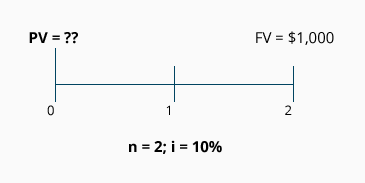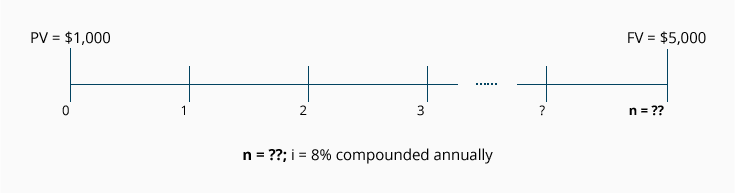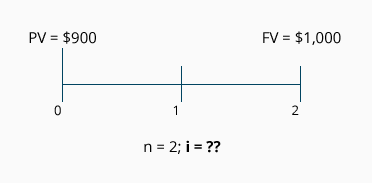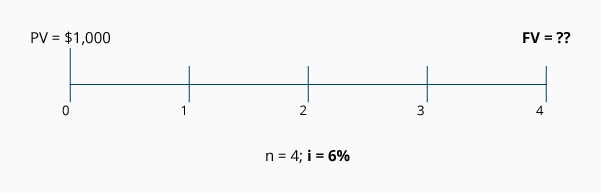## Introduction to the Present Value of a Single Amount (PV)

If you received \$100 today and deposited it into a savings account, it would grow over time to be worth more than \$100. This fact of financial life is a result of the time value of money, a concept which says it's more valuable to receive \$100 now rather than a year from now. To put it another way, the present value of receiving \$100 one year from now is less than \$100.

Accountants use Present Value (PV) calculations to account for the time value of money in a number of different applications. For example, assume your company provides a service in December 2022 and agrees to be paid \$100 in December 2023. The time value of money tells us that the part of the \$100 is interest you will earn for waiting one year for the \$100. Perhaps only \$91 of the \$100 is service revenue earned in 2022 and \$9 is interest that will be earned in 2023. The calculation of present value will remove the interest, so that the amount of the service revenue can be determined. Another example might involve the purchase of land: the owners will either sell it to you for \$160,000 today, or for \$200,000 if you pay at the end of two years. To help analyze the alternatives, you would use a PV calculation to tell you the interest rate implicit in the second option.

PV calculations can also tell you such things as how much money to invest right now in return for specific cash amounts to be received in the future, or how to estimate the rate of return on your investments. Our focus will be on single amounts that are received or paid in the future. We'll discuss PV calculations that solve for the present value, the implicit interest rate, and/or the length of time between the present and future amounts.

## Calculations for the Present Value of a Single Amount

At the outset, it's important for you to understand that PV calculations involve cash amounts—not accrual amounts.

In present value calculations, future cash amounts are discounted back to the present time. (Discounting means removing the interest that is imbedded in the future cash amounts.) As a result, present value calculations are often referred to as a discounted cash flow technique.

PV calculations involve the compounding of interest. This means that any interest earned is reinvested and itself will earn interest at the same rate as the principal. In other words, you "earn interest on interest." The compounding of interest can be very significant when the interest rate and/or the number of years is sizeable.

We will use present value (PV) to mean a single future amount such as one receipt or one payment. Here are the components of a present value (PV) calculation:

1. Present value amount (PV)
2. Future value amount (FV)
3. Length of time before the future value amount occurs (n)
4. Interest rate used for discounting the future value amount (i)

If you know any three of these four components, you will be able to calculate the unknown component. Accountants are often called upon to calculate this unknown component.

## Visualizing the Present Value (PV) Amount

Let's assume that Customer X provides your company with a promissory note for \$1,000 in exchange for service your company provided. The note is due at the end of two years and it does not specify any interest. The fair market value of the note and the fair market value of the service are not known. Because of the time value of money, you know that some interest is involved in a two-year note, even though it is not stated explicitly. You estimate the interest rate by considering both the length of the loan and the credit worthiness of Customer X. If Customer X is a reputable company like Google, you know there is minimal risk and a low interest rate would be used. If, however, Customer X has a bad credit history, then a high interest rate would be used. Let's assume that you have determined 10% to be the appropriate rate for Customer X. We now know three of the four components we need: (1) the future value amount (\$1,000), (2) the length of time (2 years), and (3) the interest rate (10%). With these three components, we know enough to calculate the fourth component, present value.

A timeline can help us visualize what is known and what needs to be computed. The present time is noted with a "0," the end of the first period is noted with a "1," and the end of the second period is noted with a "2."

The following timeline depicts the information we know, along with the unknown component, (PV):The letter "n" refers to the length of time (in this case, two years). The letter "i" refers to the percentage interest rate used to discount the future amount (in this case, 10%). Both (n) and (i) are stated within the context of time (e.g., two years at a 10% annual interest rate).

(Later on we will give examples where (n) and (i) pertain to a half-year, a quarter of a year, or a month.)

## Visualizing The Length of Time (n)

Sometimes the present value, the future value, and the interest rate for discounting are known, but the length of time before the future value occurs is unknown. To illustrate, let's assume that \$1,000 will be invested today at an annual interest rate of 8% compounded annually. The investment will be sold when its future value reaches \$5,000. Because we know three components, we can solve for the unknown fourth component—the number of years it will take for \$1,000 of present value to reach the future value of \$5,000.

The following timeline depicts the known components and the unknown component (n):## Visualizing The Interest Rate (i)

What will our timeline look like when our unknown component is the interest rate? For this example, let's assume that we know the following: the present value is \$900, the future value amount is \$1,000, and the length of time before the future value occurs is two years. Since we know three of the components, the fourth one—the interest rate that will discount the future value amount to the present value—can be calculated.

The following timeline depicts the known components and the unknown component (i):## Visualizing The Future Value Amount (FV)

Let's assume that the interest rate, the length of time, and the present value are known, and the future value is the component we don't know. If a present value of \$1,000 is invested at 6% per year and compounded annually for four years, what is the future value amount?

The following timeline depicts the known components and the unknown component (FV):As illustrated in the four timelines above, there is a logical connection between present value and future value. (Learn more about Future Value of a Single Amount.)